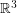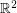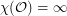# geometric graph

## Circular colouring the orthogonality graph ★★

Author(s): DeVos; Ghebleh; Goddyn; Mohar; Naserasr

Letdenote the graph with vertex set consisting of all lines through the origin inand two vertices adjacent inif they are perpendicular.

Problem   Is?

## Coloring the Odd Distance Graph ★★★

Author(s): Rosenfeld

The Odd Distance Graph, denoted, is the graph with vertex setand two points adjacent if the distance between them is an odd integer.

Question   Is?

Keywords: coloring; geometric graph; odd distance

## Universal point sets for planar graphs ★★★

Author(s): Mohar

We say that a setis-universal if everyvertex planar graph can be drawn in the plane so that each vertex maps to a distinct point in, and all edges are (non-intersecting) straight line segments.

Question   Does there exist an-universal set of size?

Keywords: geometric graph; planar graph; universal set